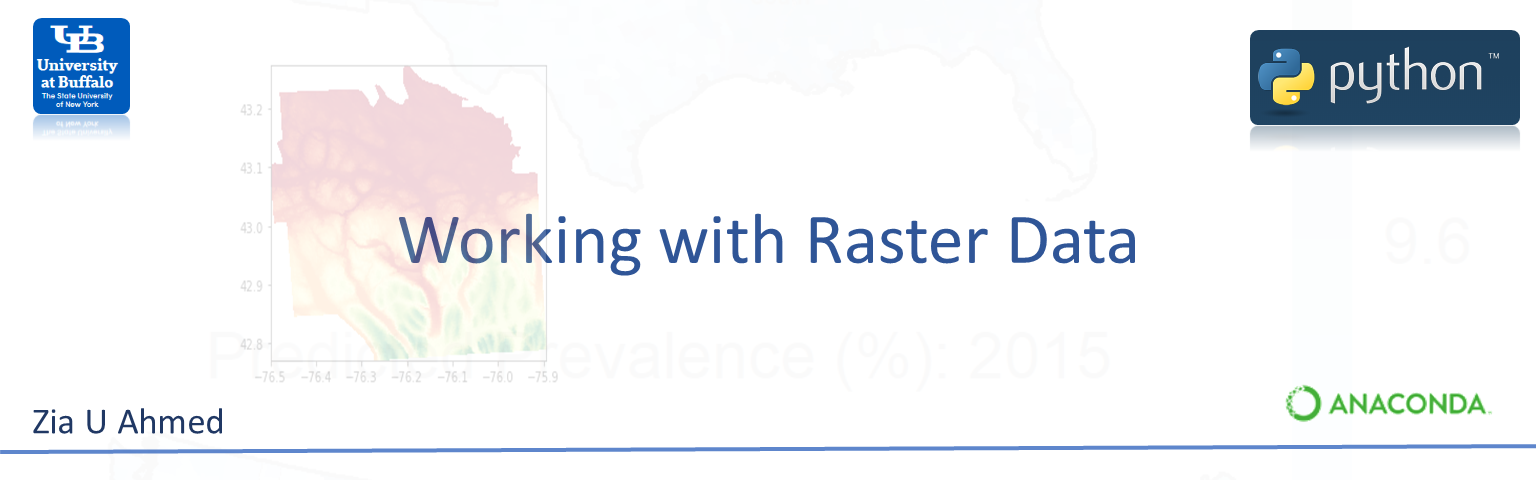# Raster Data¶

Unlike vector data, a raster data consists of cells or pixels organized into rows and columns as a matrix where each cell contains a value representing geographical feature on the earth. The size of the area on the surface that each pixel covers is known as the spatial resolution of the image. For instance, an image that has a 1 m spatial resolution means that each pixel in the image represents a 1 m x 1 m area. There are two types of raster data: continuous and discrete. An example of discrete raster data is Land use raster. Data types are flexible, including discrete and categorical data, such as soil or land-use maps, or continuous data as in digital elevation models, precipitation gradient maps, or pollutant concentration maps, etc.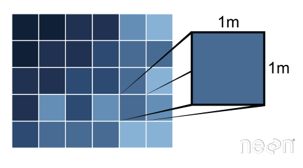Generally, two types of raster use in GIS and remote sensing application: a single band, or layer measure of a single characteristic of spatial area and multiple bands raster contains multiple spatially corresponding matrices of cell values representing the same spatial area. An example of a single-band raster data set is a digital elevation model (DEM). Each cell in a DEM contains only one value representing elevation of earth surface. Most satellite imagery has multiple bands, typically containing values within band of the electromagnetic spectrum.

In this exercise, we will use SRTM 90 Digital Elevation Model of Onondaga County, New York State which was downloaded from CGIAR-CSI.

The data set use in this exercise could be found here.

• 90 m SRTM DEM of Onondaga county, New York State
• Landsat8 multibands Images
• DEM for 4 Great Plain States
• A Spatial Point Data frame

### Set working directory¶

In :
import os
path= "E:/GitHub/geospatial-python-github.io/Lesson_06_working_with_raster_data/"
os.chdir(path)
print("Current Working Directory " , os.getcwd())


In :
%matplotlib inline

import sys
from glob import glob
from osgeo import ogr, gdal
from osgeo import gdalconst
import subprocess

import pandas as pd

import matplotlib.pyplot as plt
from matplotlib.patches import Patch
from matplotlib.colors import ListedColormap, BoundaryNorm
import numpy as np

import seaborn as sns

import geopandas as gpd
import pycrs
import fiona
from fiona.crs import from_epsg
from shapely.geometry import box
from shapely.geometry import Point
import shapely.geometry as geoms

import rasterio as rio
from rasterio.plot import show
import rasterio.warp
import rasterio.shutil
from rasterio.warp import calculate_default_transform, reproject, Resampling
from rasterio.plot import plotting_extent
from rasterio.plot import show_hist
from rasterio.merge import merge
from rasterio import Affine, MemoryFile
from rasterio.enums import Resampling
from rasterio import plot

import rasterstats as rs
import georasters as gr
from rastertodataframe import raster_to_dataframe

import earthpy.spatial as es
import earthpy.plot as ep
import earthpy as et

from sklearn.model_selection import train_test_split
from sklearn.linear_model import LinearRegression
from sklearn import metrics
from sklearn.model_selection import cross_val_score
from sklearn.model_selection import KFold
from sklearn.model_selection import cross_val_predict
from sklearn.model_selection import cross_validate

C:\anaconda3\envs\tensorflow\lib\site-packages\pysal\explore\segregation\network\network.py:16: UserWarning: You need pandana and urbanaccess to work with segregation's network module
You can install them with  pip install urbanaccess pandana or conda install -c udst pandana urbanaccess
C:\anaconda3\envs\tensorflow\lib\site-packages\pysal\model\spvcm\abstracts.py:10: UserWarning: The dill module is required to use the sqlite backend fully.

In :
print(f"Python {sys.version}")
print(f"geopandas {gpd.__version__}")
print(f"osgeo {gdal.__version__}")
print(f"rasterio {rio.__version__}")

Python 3.7.6 | packaged by conda-forge | (default, Jan  7 2020, 21:48:41) [MSC v.1916 64 bit (AMD64)]
geopandas 0.6.2
osgeo 3.0.2
rasterio 1.1.2


### Setting consistent plotting style throughout notebook¶

In :
sns.set_style("white")
sns.set(font_scale=1.5)


# Read and Write Raster Data¶

In this exercise, we will use SRTM 90 Digital Elevation Model of Onondaga County, New York State which was downloaded from CGIAR-CSI. We will use rasterio package to open and visualization raster in Python.

### Open With Rasterio¶

In :
dem_path = "Data_06/RASTER/DEM/Onondaga_DEM.tif"
dem=rio.open(dem_path)


#### Pojection¶

In :
dem.crs

Out:
CRS.from_epsg(4326)

#### Raster width¶

In :
dem.width

Out:
534

#### Raster hieight¶

In :
# raster column
dem.height

Out:
443

#### Number of raster bands¶

In :
dem.count

Out:
1

#### Data type of the values¶

In :
type(dem)

Out:
rasterio.io.DatasetReader

#### Raster Meta-data¶

In :
DEM_meta=dem.meta
print(DEM_meta)

{'driver': 'GTiff', 'dtype': 'float32', 'nodata': -3.4028230607370965e+38, 'width': 534, 'height': 443, 'count': 1, 'crs': CRS.from_epsg(4326), 'transform': Affine(0.0011330640324679006, 0.0, -76.50012580544984,
0.0, -0.00113306403246791, 43.27333404698799)}


#### Simple raster plot¶

In :
show(dem,cmap='RdYlGn')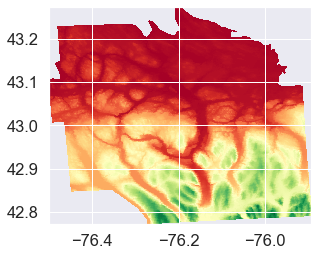Out:
<matplotlib.axes._subplots.AxesSubplot at 0x15bec2875c8>

#### Raster Histogram¶

In :
from rasterio.plot import show_hist
show_hist(dem, bins=20, lw=0.0, stacked=False, alpha=0.3,
histtype='stepfilled', title="Histogram")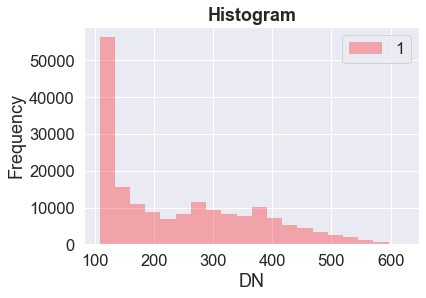### Open with GDAL¶

You can also open a raster layer with gdal.Open()

In :
raster = gdal.Open(dem_path)


#### Check type of the variable 'raster'¶

In :
type(raster)

Out:
osgeo.gdal.Dataset

#### Raster properties¶

In :
gt =raster.GetGeoTransform()
print (gt)

(-76.50012580544984, 0.0011330640324679006, 0.0, 43.27333404698799, 0.0, -0.00113306403246791)


#### Raster Resolution¶

In :
pixelSizeX = gt
pixelSizeY =-gt
print (pixelSizeX)
print (pixelSizeY)

0.0011330640324679006
0.00113306403246791


#### Projection¶

In :
raster.GetProjection()

Out:
'GEOGCS["WGS 84",DATUM["WGS_1984",SPHEROID["WGS 84",6378137,298.257223563,AUTHORITY["EPSG","7030"]],AUTHORITY["EPSG","6326"]],PRIMEM["Greenwich",0,AUTHORITY["EPSG","8901"]],UNIT["degree",0.0174532925199433,AUTHORITY["EPSG","9122"]],AXIS["Latitude",NORTH],AXIS["Longitude",EAST],AUTHORITY["EPSG","4326"]]'

#### Plot raster¶

In :
data = raster.GetRasterBand(1).ReadAsArray()
plt.imshow(data,cmap='gray')
plt.show()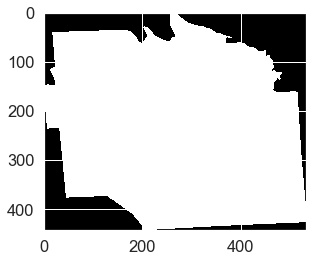## Write raster data¶

### With Rasterio¶

We can export a raster file in python using the rasterio write() function.

Use rio.open() to create a new blank raster ‘template’. Then write the DEM numpy array to to that template using dst.write().

In :
outTIF= "Data_06/RASTER/DEM/Onondaga_DEM_NEW.tif"
dem_meta=dem.profile
with rio.open(outTIF, 'w', **dem_meta) as dst:

In :
src = rio.open("Data_06/RASTER/DEM/Onondaga_DEM_NEW.tif")
show(src)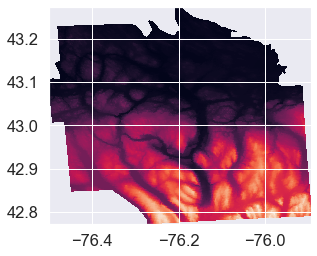Out:
<matplotlib.axes._subplots.AxesSubplot at 0x15bef3660c8>

### Write with GDAL¶

Now I will show how to write raster with GDAL driver. We will use a method to save the data in a format provided by the user.

In :
g = gdal.Open ("Data_06/RASTER/DEM/Onondaga_DEM_NEW.tif" )

In :
# Get the projection information
g.GetProjection()

Out:
'GEOGCS["WGS 84",DATUM["WGS_1984",SPHEROID["WGS 84",6378137,298.257223563,AUTHORITY["EPSG","7030"]],AUTHORITY["EPSG","6326"]],PRIMEM["Greenwich",0,AUTHORITY["EPSG","8901"]],UNIT["degree",0.0174532925199433,AUTHORITY["EPSG","9122"]],AXIS["Latitude",NORTH],AXIS["Longitude",EAST],AUTHORITY["EPSG","4326"]]'
In :
# Get the x, y and number of bands from the original file
x_size, y_size, n_bands = g.RasterXSize, g.RasterYSize, g.RasterCount
# Get a handler to a driver
driver = gdal.GetDriverByName ( "HFA" )
data

Out:
array([[-3.402823e+38, -3.402823e+38, -3.402823e+38, ..., -3.402823e+38,
-3.402823e+38, -3.402823e+38],
[-3.402823e+38, -3.402823e+38, -3.402823e+38, ..., -3.402823e+38,
-3.402823e+38, -3.402823e+38],
[-3.402823e+38, -3.402823e+38, -3.402823e+38, ..., -3.402823e+38,
-3.402823e+38, -3.402823e+38],
...,
[-3.402823e+38, -3.402823e+38, -3.402823e+38, ..., -3.402823e+38,
-3.402823e+38, -3.402823e+38],
[-3.402823e+38, -3.402823e+38, -3.402823e+38, ..., -3.402823e+38,
-3.402823e+38, -3.402823e+38],
[-3.402823e+38, -3.402823e+38, -3.402823e+38, ..., -3.402823e+38,
-3.402823e+38, -3.402823e+38]], dtype=float32)
In :
# Next line creates the output dataset with
# 1. The filename ("Onondaga_DEM.img")
# 2. The raster size (x_size, y_size)
# 3. The number of bands
# 4.The data type (in this case, Byte.
#  Other typical values might be gdal.GDT_Int16
#  or gdal.GDT_Float32)
dataset_out = driver.Create( "Data_06/RASTER/DEM/Onondaga_DEM_gdal.img", x_size, y_size, n_bands, \
gdal.GDT_Byte )

In :
# Set the output geotransform by reading the input one
dataset_out.SetGeoTransform ( g.GetGeoTransform() )

# Set the output projection by reading the input one
dataset_out.SetProjection ( g.GetProjectionRef() )

# Now, get band # 1, and write our data array.
# Note that the data array needs to have the same type
# as the one specified for dataset_out
dataset_out.GetRasterBand ( 1 ).WriteArray ( data )

# This bit forces GDAL to close the file and write to it
dataset_out = None

In :
!gdalinfo "Data_06/RASTER/DEM/Onondaga_DEM_gdal.img"

Driver: HFA/Erdas Imagine Images (.img)
Files: Data_06/RASTER/DEM/Onondaga_DEM_gdal.img
Size is 534, 443
Coordinate System is:
BOUNDCRS[
SOURCECRS[
GEOGCRS["WGS 84",
DATUM["World Geodetic System 1984",
ELLIPSOID["WGS 84",6378137,298.257223563,
LENGTHUNIT["metre",1]]],
PRIMEM["Greenwich",0,
ANGLEUNIT["degree",0.0174532925199433]],
CS[ellipsoidal,2],
AXIS["geodetic latitude (Lat)",north,
ORDER,
ANGLEUNIT["degree",0.0174532925199433]],
AXIS["geodetic longitude (Lon)",east,
ORDER,
ANGLEUNIT["degree",0.0174532925199433]],
ID["EPSG",4326]]],
TARGETCRS[
GEOGCRS["WGS 84",
DATUM["World Geodetic System 1984",
ELLIPSOID["WGS 84",6378137,298.257223563,
LENGTHUNIT["metre",1]]],
PRIMEM["Greenwich",0,
ANGLEUNIT["degree",0.0174532925199433]],
CS[ellipsoidal,2],
AXIS["latitude",north,
ORDER,
ANGLEUNIT["degree",0.0174532925199433]],
AXIS["longitude",east,
ORDER,
ANGLEUNIT["degree",0.0174532925199433]],
ID["EPSG",4326]]],
ABRIDGEDTRANSFORMATION["Transformation from WGS 84 to WGS84",
METHOD["Position Vector transformation (geog2D domain)",
ID["EPSG",9606]],
PARAMETER["X-axis translation",0,
ID["EPSG",8605]],
PARAMETER["Y-axis translation",0,
ID["EPSG",8606]],
PARAMETER["Z-axis translation",0,
ID["EPSG",8607]],
PARAMETER["X-axis rotation",0,
ID["EPSG",8608]],
PARAMETER["Y-axis rotation",0,
ID["EPSG",8609]],
PARAMETER["Z-axis rotation",0,
ID["EPSG",8610]],
PARAMETER["Scale difference",1,
ID["EPSG",8611]]]]
Data axis to CRS axis mapping: 2,1
Origin = (-76.500125805449841,43.273334046987991)
Pixel Size = (0.001133064032468,-0.001133064032468)
Corner Coordinates:
Upper Left  ( -76.5001258,  43.2733340) ( 76d30' 0.45"W, 43d16'24.00"N)
Lower Left  ( -76.5001258,  42.7713867) ( 76d30' 0.45"W, 42d46'16.99"N)
Upper Right ( -75.8950696,  43.2733340) ( 75d53'42.25"W, 43d16'24.00"N)
Lower Right ( -75.8950696,  42.7713867) ( 75d53'42.25"W, 42d46'16.99"N)
Center      ( -76.1975977,  43.0223604) ( 76d11'51.35"W, 43d 1'20.50"N)
Band 1 Block=64x64 Type=Byte, ColorInterp=Undefined
Description = Layer_1
LAYER_TYPE=athematic



# Working with Multi Band Raster¶

For loading multi-bands image in Python, we will use a sub-set of Landsat 8 multispectral image covering Onondaga county of New York state. This image was downloaded from USGS Earth Explore.

#### Open individual bands (b2 to b4) one by one¶

In :
b2="Data_06/RASTER/LC08_20150706_subset/LC08_L1TP_015030_20150716_20170226_01_T1_B2.TIF"
b3="Data_06/RASTER/LC08_20150706_subset/LC08_L1TP_015030_20150716_20170226_01_T1_B3.TIF"
b4="Data_06/RASTER/LC08_20150706_subset/LC08_L1TP_015030_20150716_20170226_01_T1_B4.TIF"
b5="Data_06/RASTER/LC08_20150706_subset/LC08_L1TP_015030_20150716_20170226_01_T1_B5.TIF"

b2_r=rio.open(b2)
b3_r=rio.open(b3)
b4_r=rio.open(b4)
b5_r=rio.open(b5)


#### Plot all four bands¶

In :
# Initialize subplots
import matplotlib.pyplot as plt
%matplotlib inline

fig, (ax1, ax2, ax3, ax4) = plt.subplots(ncols=4, nrows=1, figsize=(10, 4), sharey=True)

# Plot 4 bands
show(b2_r, ax=ax1)
show(b3_r, ax=ax2)
show(b4_r, ax=ax3)
show(b5_r, ax=ax4)

ax1.set_title("Band-2")
ax2.set_title("Band-3")
ax3.set_title("Band-4")
ax4.set_title("Band-5")

Out:
Text(0.5, 1.0, 'Band-5')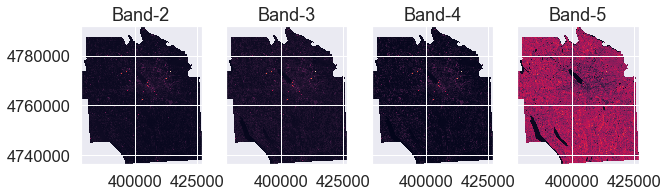## Stack Multi Band Imagery¶

Some remote sensing datasets are stored with each band in a separate file. However, often you want to use all of the bands together in your analysis. For example you need all of the bands together in the same file or “stack” in order to plot a color RGB image. EarthPy has a stack() function that allows you to take a set of .tif files that are all in the same spatial extent, CRS and resolution and either export them together a single stacked .tif file or work with them in Python directly as a stacked numpy array.

To begin using the EarthPy stack() function, import the needed packages and create an array to be plotted. Below you plot the data as continuous with a colorbar using the plot_bands() function.

We will use es.stack() function of earthpy library to create a raster stack of multi-band raster. It need three steps:

1. Create a raster list using glob() function
2. Create a path and define a name for mutli-band raster
3. Apply es.stack() to creat new stacked raster with all bands save as multi tif
4. Then apply rio.open to read the raster bands

#### Creat a Raster List¶

You will use the glob function see the files in landsat data directory

In :
glob ("Data_06/RASTER/LC08_20150706_subset/*")

Out:
['Data_06/RASTER/LC08_20150706_subset\\landsat_multi.tif',
'Data_06/RASTER/LC08_20150706_subset\\LC08_L1TP_015030_20150716_20170226_01_T1_ANG.txt',
'Data_06/RASTER/LC08_20150706_subset\\LC08_L1TP_015030_20150716_20170226_01_T1_B1.TIF',
'Data_06/RASTER/LC08_20150706_subset\\LC08_L1TP_015030_20150716_20170226_01_T1_B10.TIF',
'Data_06/RASTER/LC08_20150706_subset\\LC08_L1TP_015030_20150716_20170226_01_T1_B11.TIF',
'Data_06/RASTER/LC08_20150706_subset\\LC08_L1TP_015030_20150716_20170226_01_T1_B2.TIF',
'Data_06/RASTER/LC08_20150706_subset\\LC08_L1TP_015030_20150716_20170226_01_T1_B3.TIF',
'Data_06/RASTER/LC08_20150706_subset\\LC08_L1TP_015030_20150716_20170226_01_T1_B4.TIF',
'Data_06/RASTER/LC08_20150706_subset\\LC08_L1TP_015030_20150716_20170226_01_T1_B5.TIF',
'Data_06/RASTER/LC08_20150706_subset\\LC08_L1TP_015030_20150716_20170226_01_T1_B6.TIF',
'Data_06/RASTER/LC08_20150706_subset\\LC08_L1TP_015030_20150716_20170226_01_T1_B7.TIF',
'Data_06/RASTER/LC08_20150706_subset\\LC08_L1TP_015030_20150716_20170226_01_T1_B8.TIF',
'Data_06/RASTER/LC08_20150706_subset\\LC08_L1TP_015030_20150716_20170226_01_T1_B9.TIF',
'Data_06/RASTER/LC08_20150706_subset\\LC08_L1TP_015030_20150716_20170226_01_T1_BQA.TIF']

Now we will create a list for band (2,3,4 and 5)

In :
multi_bands=glob( "Data_06/RASTER/LC08_20150706_subset/*B[2:3:4:5]*.tif")
multi_bands

Out:
['Data_06/RASTER/LC08_20150706_subset\\LC08_L1TP_015030_20150716_20170226_01_T1_B2.TIF',
'Data_06/RASTER/LC08_20150706_subset\\LC08_L1TP_015030_20150716_20170226_01_T1_B3.TIF',
'Data_06/RASTER/LC08_20150706_subset\\LC08_L1TP_015030_20150716_20170226_01_T1_B4.TIF',
'Data_06/RASTER/LC08_20150706_subset\\LC08_L1TP_015030_20150716_20170226_01_T1_B5.TIF']

Now you have a list of all of the landsat bands in your landsat collections folder. You could chose to open each file individually using the rio.open (rasterio library) function.

Remember that Python uses 0 based indexing so band 3 is actually at index  not .

In :
with rio.open(multi_bands) as src: